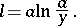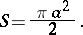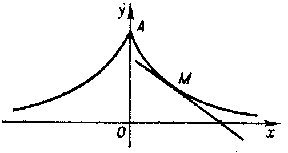# Tractrix

A plane transcendental curve whose equation in rectangular Cartesian coordinates has the formThe tractrix is symmetric about the origin (see Fig.), the-axis being an asymptote. The pointis a cusp with vertical tangent. The length of the arc measured from the pointis:The radius of curvature is:The area bounded by the tractrix and its asymptote is:Figure: t093570a

The rotation of the tractrix around the-axis generates a pseudo-sphere. The length of the tangent, that is, of the segment between the point of tangencyand the-axis, is constant. This property enables one to regard the tractrix as the trajectory of the end of a line segment of length, when the other end moves along the-axis. The notion of a tractrix generalizes to the case when the end of the segment does not move along a straight line, but along some given curve; the curve obtained in this way is called the trajectory of the given curve.